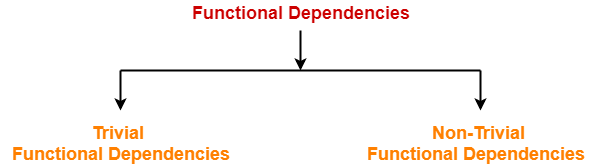# Functional Dependency in DBMS

## Functional Dependency-

 In any relation, a functional dependency α → β holds if- Two tuples having same value of attribute α also have same value for attribute β.

Mathematically,

If α and β are the two sets of attributes in a relational table R where-

• α ⊆ R
• β ⊆ R

Then, for a functional dependency to exist from α to β,

If t1[α] = t2[α], then t1[β] = t2[β]

 α β t1[α] t1[β] t2[α] t2[β] ……. …….

 fd : α → β

## Types Of Functional Dependencies-

There are two types of functional dependencies-1. Trivial Functional Dependencies
2. Non-trivial Functional Dependencies

## 1. Trivial Functional Dependencies-

• A functional dependency X → Y is said to be trivial if and only if Y ⊆ X.
• Thus, if RHS of a functional dependency is a subset of LHS, then it is called as a trivial functional dependency.

### Examples-

The examples of trivial functional dependencies are-

• AB → A
• AB → B
• AB → AB

## 2. Non-Trivial Functional Dependencies-

• A functional dependency X → Y is said to be non-trivial if and only if Y ⊄ X.
• Thus, if there exists at least one attribute in the RHS of a functional dependency that is not a part of LHS, then it is called as a non-trivial functional dependency.

### Examples-

The examples of non-trivial functional dependencies are-

• AB → BC
• AB → CD

## Inference Rules-

### Reflexivity-

If B is a subset of A, then A → B always holds.

### Transitivity-

If A → B and B → C, then A → C always holds.

### Augmentation-

If A → B, then AC → BC always holds.

### Decomposition-

If A → BC, then A → B and A → C always holds.

### Composition-

If A → B and C → D, then AC → BD always holds.

If A → B and A → C, then A → BC always holds.

## Rules for Functional Dependency-

### Rule-01:

A functional dependency X → Y will always hold if all the values of X are unique (different) irrespective of the values of Y.

### Example-

Consider the following table-

 A B C D E 5 4 3 2 2 8 5 3 2 1 1 9 3 3 5 4 7 3 3 8

The following functional dependencies will always hold since all the values of attribute ‘A’ are unique-

• A → B
• A → BC
• A → CD
• A → BCD
• A → DE
• A → BCDE

In general, we can say following functional dependency will always hold-

 A → Any combination of attributes A, B, C, D, E

Similar will be the case for attributes B and E.

### Rule-02:

A functional dependency X → Y will always hold if all the values of Y are same irrespective of the values of X.

### Example-

Consider the following table-

 A B C D E 5 4 3 2 2 8 5 3 2 1 1 9 3 3 5 4 7 3 3 8

The following functional dependencies will always hold since all the values of attribute ‘C’ are same-

• A → C
• AB → C
• ABDE → C
• DE → C
• AE → C

In general, we can say following functional dependency will always hold true-

 Any combination of attributes A, B, C, D, E → C

Combining Rule-01 and Rule-02 we can say-

 In general, a functional dependency α → β always holds- If either all values of α are unique or if all values of β are same or both.

### Rule-03:

For a functional dependency X → Y to hold, if two tuples in the table agree on the value of attribute X, then they must also agree on the value of attribute Y.

### Rule-04:

For a functional dependency X → Y, violation will occur only when for two or more same values of X, the corresponding Y values are different.

Next Article- Equivalence of Functional Dependencies

Get more notes and other study material of Database Management System (DBMS).

Watch video lectures by visiting our YouTube channel LearnVidFun.

SummaryArticle Name
Functional Dependency in DBMS
Description
In DBMS, a functional dependency α → β holds if two tuples having same value of attribute α have same value for attribute β. Examples of Trivial and Non-Trivial Functional Dependency.
Author
Publisher Name
Gate Vidyalay
Publisher Logo